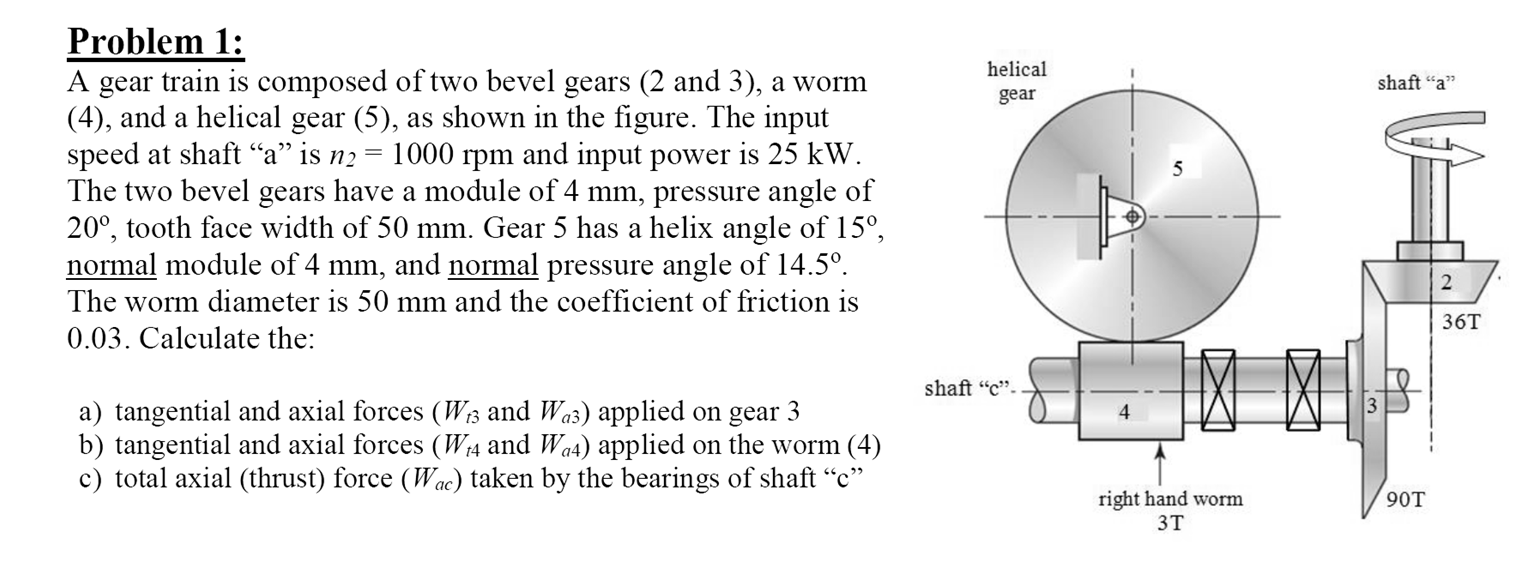### Create an Account

Home / Questions / A gear train is composed of two bevel gears 2 and 3 a worm 4 and a helical gear as shown ...

# A gear train is composed of two bevel gears 2 and 3 a worm 4 and a helical gear as shown in the figure The input speed at shaft a is n2 1000 rpm and input power is 25 kW The two

A gear train is composed of two bevel gears (2 and 3), a worm (4), and a helical gear (5), as shown in the figure. The input speed at shaft "a" is n2 = 1000 rpm and input power is 25 kW. The two bevel gears have a module of 4 mm, pressure angle of 200, tooth face width of 50 mm. Gear 5 has a helix angle of 15A????1, normal module of 4 mm, and normal pressure angle of 14.5A????1. The worm diameter is 50 mm and the coefficient of friction is 0.03. Calculate the:

a) tangential and axial forces (Wt.; and W03) applied on gear 3

b) tangential and axial forces ( W4 and W04) applied on the worm (4)

c) total axial (thrust) force (W(,) taken by the bearings of shaft "c"Show transcribed image text A gear train is composed of two bevel gears (2 and 3), a worm (4), and a helical gear (5), as shown in the figure. The input speed at shaft a is n2 = 1000 rpm and input power is 25 kW. The two bevel gears have a module of 4 mm, pressure angle of 20 degree, tooth face width of 50 mm. Gear 5 has a helix angle of 15 degree, normal module of 4 mm, and normal pressure angle of 14.5 degree. The worm diameter is 50 mm and the coefficient of friction is 0.03. Calculate the: a) tangential and axial forces (W13 and W03) applied on gear 3 b) tangential and axial forces ( W14 and W04) applied on the worm (4) c) total axial (thrust) force (Wac) taken by the bearings of shaft c

May 13 2020 View more View LessSubscribe To Get Solution